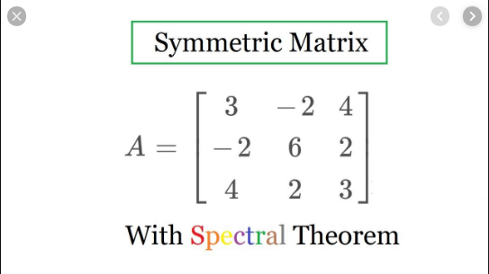# Deep Learning book in plain English Ch1

Source: Deep Learning on Medium# Deep Learning book in plain English Ch1

This story is a summary of my intuition about the Deep learning book (Ch2) by Ian Goodfellow, Yoshua Bengio, and Aaron Courville

# Vectors and Tensors

I will start by these two crucial blocks of any system, I am skipping matrices and scalar values as I think they are pretty obvious to many readers.

# Vectors

An array of numbers, we can think of as a set of coordinates having each values in a different axis for example x = [1,2], x is a vector having values in two different axes.

# Tensors

The reason why I began with vectors is actually to compare them with tensors as this comparison has always made me pretty confused. A tensor is an array having more than 2 values for example x = (1,2,3,..)

# Multiplying matrices

One of the main operations in deep learning is matrix multiplication. For example let’s consider an example of input data [0,1,2] and a dense layer of weight vector transposed([0.1, 0.2, 0.3]) (note that I added transposed as I needed to convert my row vector to column vector to be able to perform matrix multiplication with it)

This operation translates to A = XB and is performed as follows:

0*0.1 + 1*0.2 + 0.3*2 = value, this is called dot product between the row vector of our data and column vector of the weight. This what really happens when you add dense layer to your network. This differs from what is called hadamard product(element wise product), this is performed as follows:

result = [0*0.1,1*0.2,0.3*2] = [0,0.2,0.6]

# Matrices features

Matrix multiplication is not commutative,

AB != BA

However dot product between vectors is commutative,

transpose(x) * y = transpose(y)*x

# What are norms?

Sometimes we need to measure the size of a given vector so we simply use norm function to do so, norm functions are simply mapping vectors to non negative values as they are the distance from the origin pt and vector X.

One of the most used norms in machine learning is L2 norm which is called Euclidean norm and is simply computed as x * transposed(x)

Another norm function is L1 norm which is used when the difference between zeros and non zeros elements within the vector is very important to consider since it puts a big weight when values changes from zero to non zero. For example, if a value in a vector changes from 0 to 10, L1 increases by 10

Another norm function is the max norm which is just the max element in a vector.

Diagonal matrix